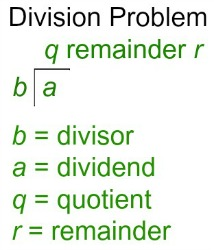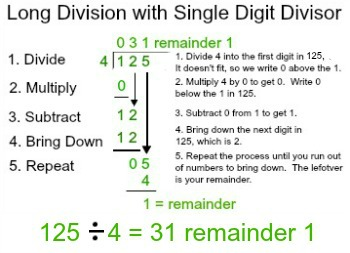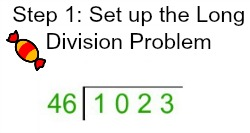# How to Divide by Double Digit Numbers

Instructor: Laura Pennington

Laura received her Master's degree in Pure Mathematics from Michigan State University. She has 15 years of experience teaching collegiate mathematics at various institutions.

This lesson will teach us how to divide by double digit numbers. We will examine the steps involved with this process, and then we will practice the process with a real world application.

## Steps To Solve

To divide by a double digit number, we use long division in the same way that we would when dividing by a single digit number. Therefore, let's start with some review of long division. First of all, let's review some vocabulary of the parts of a division problem.When we divide a by b, we call b the divisor, a the dividend, the number of times b fits into a the quotient, and the leftover amount the remainder. Now let's move onto the steps of long division when the divisor is a single digit number. Those steps are divide, multiply, subtract, bring down, and repeat until there are no more digits to bring down. To illustrate the steps, let's use the example of dividing 4 into 125.Alright, now that we've got the long division process fresh in our heads, let's extend it to double digit divisors. The main difference when we have a double-digit divisor is that when we start, we begin by dividing the divisor into the first two digits of the dividend, rather than just the first digit of the divisor. Something else that is extremely helpful when dividing by a double-digit number is to create a multiplication table displaying the divisor multiplied by the integers 1 through 9 before starting the problem. Altogether, this leads to the following steps to divide by a double digit number.

1. Set up a long division problem.
2. Make a multiplication table for the divisor times each integer from 1 to 9.
3. Carry out your long division as you would with a single digit divisor (divide, multiply, subtract, bring down, repeat). Your multiplication table will help you along.

Alright, that's not so bad! We can do this!

## Solution

To divide by a double digit number, we use the following steps.

1. Set up a long division problem.
2. Make a multiplication table for the divisor times each integer from 1 to 9.
3. Carry out your long division (divide, multiply, subtract, bring down, repeat), starting by dividing the divisor into the first two digits of the dividend. Your multiplication table will help you along.

## Application

Now that we know the steps involved in dividing by a double digit number, let's put those steps into practice with an application. Suppose you are in a class of 46 students. Your teacher has a large bag of candy that she plans to hand out throughout the school year that has 1023 pieces of candy in it. She tells the class that if someone can figure out how many pieces of candy each student will get throughout the year if she splits the candy up equally between the 46 students and how much candy will be left over, then that person will get the leftover candy today (as well as their fair share throughout the year).Hmm…so we want to know how many pieces of candy each of the 46 students gets if the 1023 pieces of candy are divided equally among them and how much is left over. Ah! This problem is asking for the quotient and remainder if we divide 1023 by 46. Great! This is a perfect opportunity to practice dividing by a double digit number!

Our first step is to set up the long division problem with 46 as our divisor and 1023 as our dividend.Next step is to create our multiplication table displaying 46 times each of the integers from 1 to 9.

1×46 = 46

2×46 = 92

3×46 = 138

4×46 = 184

5×46 = 230

6×46 = 276

7×46 = 322

8×46 = 368

9×46 = 414

To unlock this lesson you must be a Study.com Member.

### Register to view this lesson

Are you a student or a teacher?

#### See for yourself why 30 million people use Study.com

##### Become a Study.com member and start learning now.
Back
What teachers are saying about Study.com

### Earning College Credit

Did you know… We have over 200 college courses that prepare you to earn credit by exam that is accepted by over 1,500 colleges and universities. You can test out of the first two years of college and save thousands off your degree. Anyone can earn credit-by-exam regardless of age or education level.